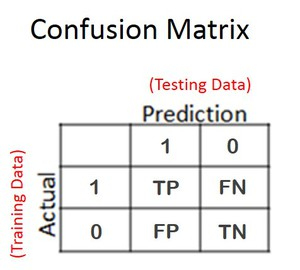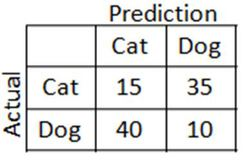# Understanding data science basics - 7.3

## Machine Learning

Version
7.3
Language
English
Product
Talend Big Data
Talend Big Data Platform
Talend Data Fabric
Talend Real-Time Big Data Platform
Module
Talend Studio
Content
Data Governance > Third-party systems > Machine Learning components
Data Quality and Preparation > Third-party systems > Machine Learning components
Design and Development > Third-party systems > Machine Learning components
This section introduces some important concepts used in machine learning.

The following concepts play a crucial role in machine learning and are part of the standard tools used by data scientists to evaluate classification models.

• Confusion Matrix: specialized table that makes it easy to visually observe classification model performance against test data where the outcomes are known (supervised learning)
• True Negative (TN): prediction equivalence to actual outcome; correct rejection
• True Positive (TP): prediction equivalence to actual outcome; correct hit
• False Negative (FN): prediction miss; erroneous rejection (Type II error)
• False Positive (FP): prediction miss; erroneous hit (Type I error)
• Accuracy: on the whole how often the classifier is correct. A = (TP+TN)/Total
• True Positive Rate (Sensitivity): TP/(TP+FN)
• True Negative Rate (Specificity): TN/(FP+TN)

Below is a generalized confusion matrix that demonstrates how it is laid out.Here is a simple but concrete example of the use of the general confusion matrix. Assume you have trained a model to analyze a series of images of cats and dogs to identify which images are cats and which are not (in this case, they are dogs). If your model is perfect, it will predict with 100% accuracy. There is also the possibility that your model results in 0% accuracy. However, the most likely outcome is somewhere in between, and this is where a confusion matrix can help.

Below is a hypothetical outcome.The hypothetical model accurately predicted 15 cat images (TP) and 10 dog, or not cat, images (TN). However, the model also falsely identified 40 dogs as cats (FN) and falsely identified 35 cats as dogs (FP).

• Accuracy of this classifier: (15+10) / (15+35+40+10) = .25
• Sensitivity of this classifier: 15/(15+35) = .3
• Specificity of this classifier: 10/(40+10) = .2

The conclusion is that this model on the whole is correct 25% of the time (accuracy). When the image is a cat, this model accurately predicts a cat 30% of the time (sensitivity). And when the image is not a cat, this model accurately predicts that it is not a cat 20% of the time (specificity).